# Fractional Delay Filters Using Farrow Structures

This example shows how to design digital fractional delay filters that are implemented using Farrow structures. Digital fractional delay filters are useful tools to fine-tune the sampling instants of signals. They are for example typically found in the synchronization of digital modems where the delay parameter varies over time. This example illustrates the Farrow structure, a popular method for implementing time-varying FIR fractional delay filters.

### Ideal Fractional Delay Filter

The ideal fractional delay filter is a linear phase allpass filter. Its impulse response is a time-shifted discrete sinc function that corresponds to a non causal filter. Since the impulse response is infinite, it cannot be made causal by a finite shift in time. It is therefore non realizable and must be approximated.

### The Farrow Structure

To compute the output of a fractional delay filter, we need to estimate the values of the input signal between the existing discrete-time samples. Special interpolation filters can be used to compute new sample values at arbitrary points. Among those, polynomial-based filters are of particular interest because a special structure - the Farrow structure - permits simple handling of coefficients. In particular, the tunability of the Farrow structure makes its well-suited for practical hardware implementations.### Maximally-Flat FIR Approximation (Lagrange Interpolation)

Lagrange interpolation is a time-domain approach that leads to a special case of polynomial-based filters. The output signal is approximated with a polynomial of degree M. The simplest case (M=1) corresponds to linear interpolation. Let's design and analyze a linear fractional delay filter that will split the unit delay by various fractions:

```Nx = 1024; Nf = 5; yw = zeros(Nx,Nf); transferFuncEstimator = dsp.TransferFunctionEstimator( ... 'SpectralAverages',25,'FrequencyRange','onesided'); arrPlotPhaseDelay = dsp.ArrayPlot('PlotType','Line','YLimits',[0 1.5], ... 'YLabel','Phase delay','SampleIncrement',1/512); arrPlotMag = dsp.ArrayPlot('PlotType','Line','YLimits',[-10 1], ... 'YLabel','Magnitude (dB)','SampleIncrement',1/512); fracDelay = dsp.VariableFractionalDelay; xw = randn(Nx,Nf); transferFuncEstimator(xw,yw); w = getFrequencyVector(transferFuncEstimator,2*pi); w = repmat(w,1,Nf); tic, while toc < 2 yw = fracDelay(xw,[0 0.2 0.4 0.6 0.8]); H = transferFuncEstimator(xw,yw); arrPlotMag(20*log10(abs(H))) arrPlotPhaseDelay(-angle(H)./w) end release(fracDelay) release(transferFuncEstimator) release(arrPlotMag)```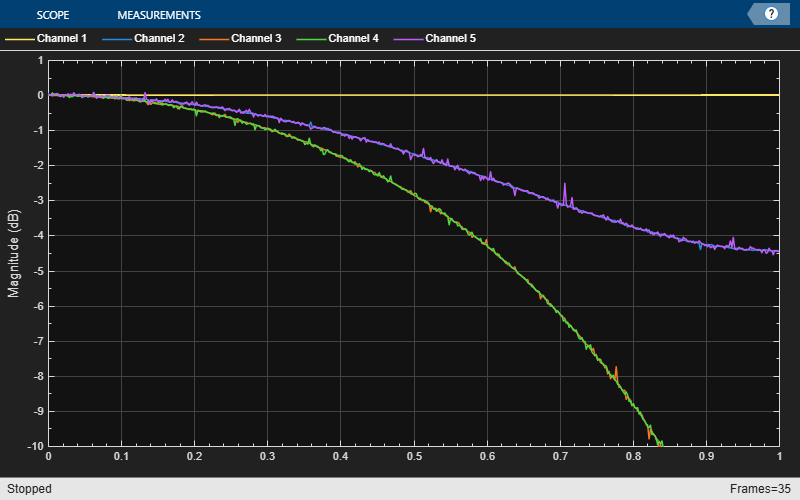`release(arrPlotPhaseDelay)`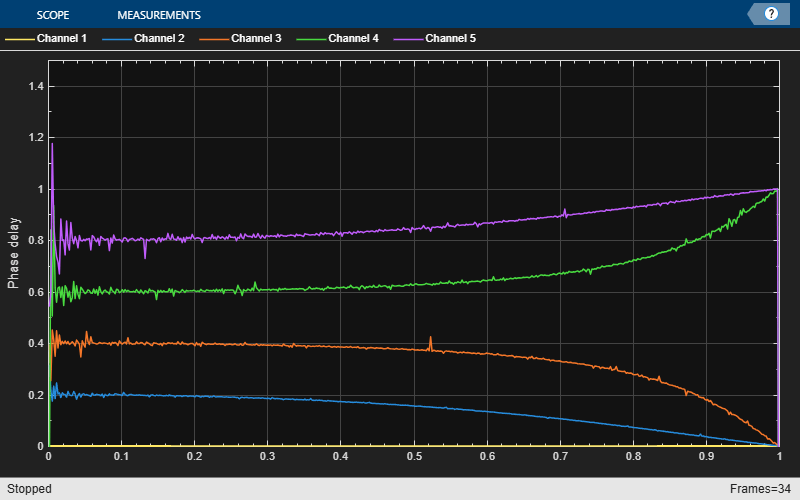For any value of the delay, the ideal filter should have both a flat magnitude response and a flat phase delay response. The approximation is correct only for the lowest frequencies. This means that in practice the signals need to be over-sampled for the linear fractional delay to work correctly. Here you apply two different fractional delays to a sine wave and use the time scope to overlay the original sine wave and the two delayed versions. A delay of 0.2 samples with a sample rate of 1000 Hz, corresponds to a delay of 0.2 ms.

```scope = timescope('SampleRate',1000, ... 'YLimits',[-1 1], ... 'TimeSpan',.02, ... 'TimeSpanOverrunAction','Scroll'); sine = dsp.SineWave('Frequency',50,'SamplesPerFrame',Nx); tic, while toc < 2 x = sine(); y = fracDelay(x,[.2 .8]); % Delay by 0.2 ms and 0.8 ms scope([x,y(:,1),y(:,2)]) end release(fracDelay) release(scope);```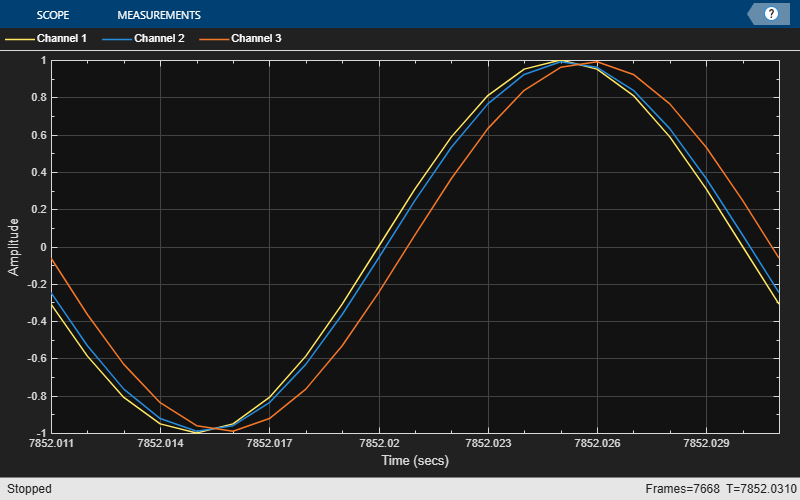Higher order Lagrange interpolators can be designed. Let's compare a cubic Lagrange interpolator with a linear one:

```farrowFracDelay = dsp.VariableFractionalDelay( ... 'InterpolationMethod','Farrow','MaximumDelay',1025); Nf = 2; yw = zeros(Nx,Nf); xw = randn(Nx,Nf); H = transferFuncEstimator(xw,yw); w = getFrequencyVector(transferFuncEstimator,2*pi); w = repmat(w,1,Nf); tic, while toc < 2 % Run for 2 seconds yw(:,1) = fracDelay(xw(:,1),0.4); % Delay by 0.4 ms yw(:,2) = farrowFracDelay(xw(:,2),1.4); % Delay by 1.4 ms H = transferFuncEstimator(xw,yw); arrPlotMag(20*log10(abs(H))) arrPlotPhaseDelay(-unwrap(angle(H))./w) end release(fracDelay) release(transferFuncEstimator) release(arrPlotMag)```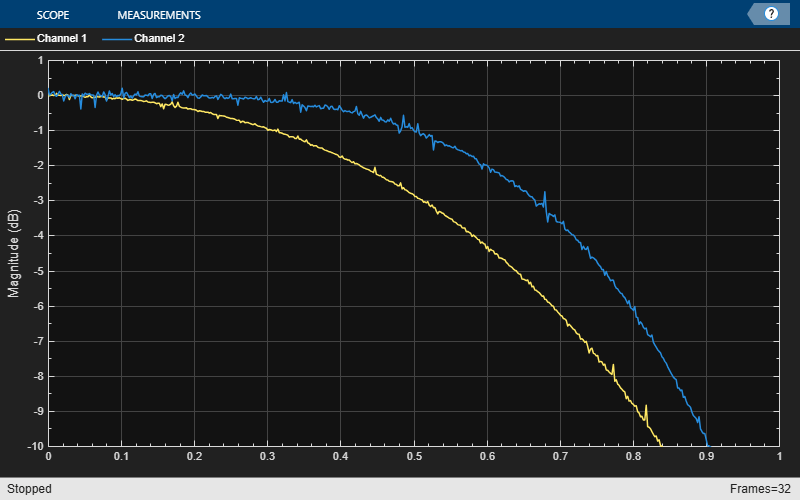`release(arrPlotPhaseDelay)`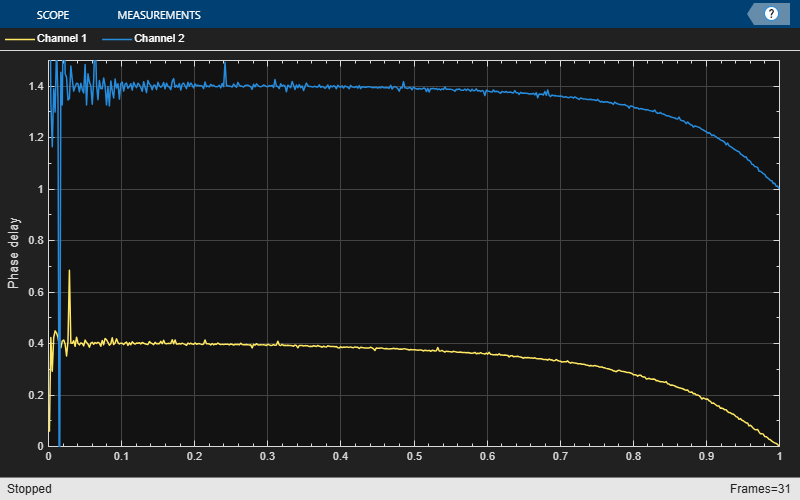Increasing the order of the polynomials slightly increases the useful bandwidth when Lagrange approximation is used. The length of the differentiating filters, that is, the number of pieces of the impulse response (number of rows of the 'Coefficients' property) is equal to the length of the polynomials (number of columns of the 'Coefficients' property). Other design methods can be used to overcome this limitation. Also notice how the phase delay of the third order filter is shifted from 0.4 to 1.4 samples at DC. Since the cubic lagrange interpolator is a 3rd order filter, the minimum delay it can achieve is 1. For this reason, the delay requested is 1.4 ms instead of 0.4 ms for this case.

```sine = dsp.SineWave('Frequency',50,'SamplesPerFrame',Nx); tic, while toc < 2 x = sine(); y1 = fracDelay(x,0.4); y2 = farrowFracDelay(x,1.4); scope([x,y1,y2]) end release(fracDelay) release(scope);```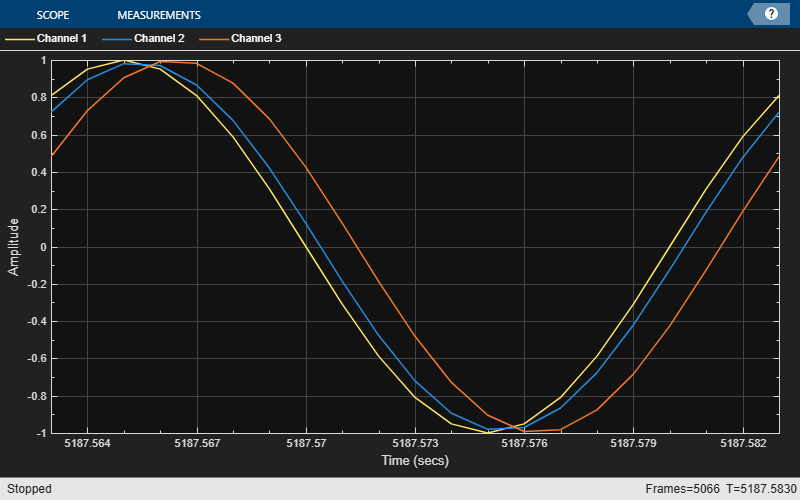### Time-Varying Fractional Delay

The advantage of the Farrow structure over a Direct-Form FIR resides in its tunability. In many practical applications, the delay is time-varying. For each new delay we would need a new set of coefficients in the Direct-Form implementation but with a Farrow implementation, the polynomial coefficients remain constant.```tic, while toc < 5 x = sine(); if toc < 1 delay = 1; elseif toc < 2 delay = 1.2; elseif toc < 3 delay = 1.4; elseif toc < 4 delay = 1.6; else delay = 1.8; end y = farrowFracDelay(x,delay); scope([x,y]) end release(fracDelay) release(scope);```